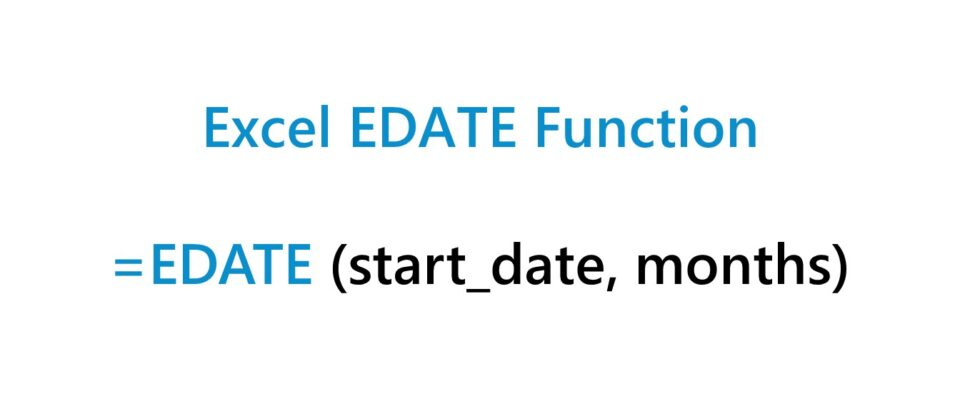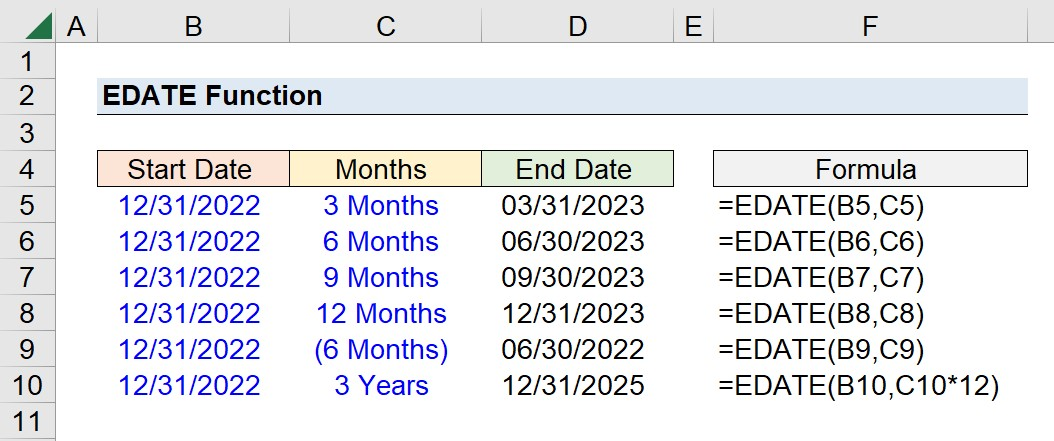Welcome to Wall Street Prep! Use code at checkout for 15% off.# EDATE Function

Guide to Understanding the Excel "EDATE" Function## How to Use EDATE Function in Excel (Step-by-Step)

The Excel EDATE function is a built-in feature that determines the specific ending date based on two user-inputs.

1. Start Date: The initial date from which the EDATE function is applied to.
2. Months: The number of months from the starting date to move either forward or backward.

Using those two inputs, the output is then the ending date.

In the context of financial modeling, the EDATE function is commonly used to return the maturity dates on debt obligations—i.e. the date on which an interest or principal payment comes due—or similar scenarios, wherein a specific event occurs on a periodic basis.

The “EOMONTH” function is also frequently used in practice. However, the difference between the EOMONTH and EDATE functions is that the former is intended to automatically return the final date in the month—as implied by the name—while the EDATE function returns a precise date that may or may not be the final date of the month.

Before utilizing the function, it is recommended to ensure that the cells are all properly formatted as dates.

By pressing the keys “Ctrl” + “1” with the date range selected, the option to format the cells as dates will then appear in the pop-up list (and the formatting style can also then be customized).

## EDATE Function Formula Syntax

The Excel EDATE function formula is as follows.

=EDATE(start_date, months)

Of the two arguments in the syntax, both are relatively intuitive.

• start_date” → The opening parameter and initial date from which the function is applied to.
• months” → The number of months to move forward or backward from the starting date.

If the “months” input is entered as a positive integer, the returned value will be on a forward basis, whereas a negative integer will be on a backward basis.

• Positive Integer → For example, entering “3” with a start date of 12/31/2022 returns an end date of 3/31/2023; the returned date is a future date relative to the starting date.
• Negative Integer → On the other hand, entering “-3” with a start date of 12/31/2022 returns an end date of 9/31/2022, i.e. the returned date is a past date before the starting date.

## EDATE Function Calculator – Excel Model Template

We’ll now move on to a modeling exercise, which you can access by filling out the form below.Submitting...

## Excel EDATE Function Exercise Example

Suppose we’re tasked with determining the end date given different monthly time intervals.

The start date remains fixed at 12/31/2022 under all six of our scenarios.

Start Date Months
12/31/2022 3 Months
12/31/2022 6 Months
12/31/2022 9 Months
12/31/2022 12 Months
12/31/2022 (6 Months)
12/31/2022 3 Years

The formula that we’ll enter for our six dates and monthly interval inputs in descending order from top to bottom are as follows.

=EDATE(B5,C5)
=EDATE(B6,C6)
=EDATE(B7,C7)
=EDATE(B8,C8)
=EDATE(B9,C9)
=EDATE(B10,C10*12)

For our fifth formula, we’ve entered the “Months” input as a negative integer; hence, a parenthesis is present around the “6 Months”.

The final scenario is also based on a yearly basis, rather than monthly. Thus, we’ll link to our “months” cells per usual, but with the added step of multiplying it by 12 to convert the units from years to months.

The screenshot below is of our completed exercise, where we can see the returned end dates given our start date and months arguments.Turbo-charge your time in Excel Used at top investment banks, Wall Street Prep's Excel Crash Course will turn you into an advanced Power User and set you apart from your peers.
Inline Feedbacks